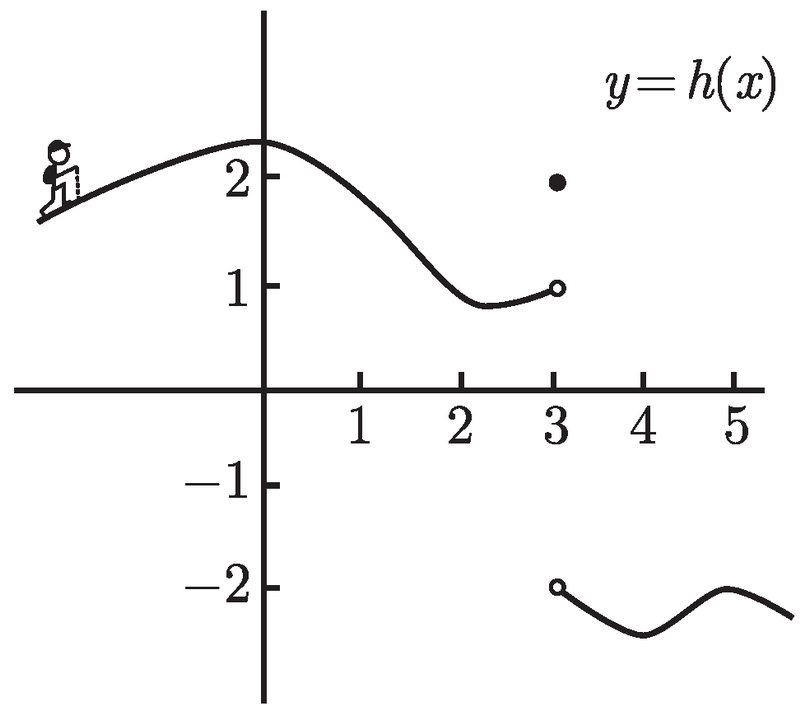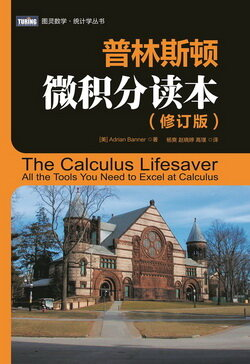# 普林斯顿微积分读本（修订版）(22)：极限导论 3.2(左极限与右极限)$\lim_{x\to3^-}h(x)=1$　及　$\lim_{x\to3^+}h(x)=-2$.

$\lim_{x\to-3}h(x),$

$\lim_{x\to a^-}f(x)=L$$\lim_{x\to a^+}f(x)=L$

$\lim_{x\to a}f(x)=L.$

$\lim_{x\to 3}h(x)$ 不存在